## The Dynamics of Galaxies Revisited.

Christian Marchal1*

1 French National Office for Aerospace Studies and Research (ONERA), BP. 72, 92322 Châtillon cedex France.

*Corresponding Author:Christian Marchal, French National Office for Aerospace Studies and Research (ONERA) BP. 72, 92322 Châtillon cedex France, Tel: +33146734040; Fax: +33146734040; E-mail: clbmarchal@wanadoo.fr

Citation: Christian Marchal (2023) The Dynamics of Galaxies Revisited. Nano Technol & Nano Sci J 5: 143.

Received: March 28, 2023; Accepted: April 14, 2023; Published: April 18, 2023.

## Abstract

The hypothesis of « Dark Matter » was invented in the thirties by Fritz Zwicky as a possible explanation of the too fast velocities of revolution of the stars in their galaxies. That hypothesis is also used for the explanation of the equilibrium of the clusters of galaxies: the visible mass of these bodies seems too small for their equilibrium. An alternative hypothesis proposed by Mordehai Milgrom is a small modification of Newton’s law of gravitation. However, a thorough analysis of pure Celestial Mechanics, without dark matter and without Milgrom’s modification, allows to explain the motion and the equilibrium of galaxies with a suitable repartition of masses (even if a part of the clouds of gas is escaping into outer space). For the clusters of galaxies, the relativistic effects are not extremely small, they are of the order of magnitude of the classical gravitational effects, and they are a part of the explanation of the equilibrium of these clusters, even if some of them are in a state of very slow dispersion (a dispersion taking billions of years). The analysis must then be made in the framework of general relativity.

## Key words

Key words: Celestial Mechanics - Dark Matter - Non-Classical Hypothesis.

## About the revolution of stars inside their galaxy.

The figure one presents eight classical examples of the dynamic of galaxies. These examples have all, more or less, the same appearance: they present two curves, the upper curve, using Doppler shift measures, gives the velocity of stars inside their galaxy in terms of the distance between the star and the center of the galaxy, the lower curve gives, in terms of that same distance, the circular velocity of equilibrium as assumed according to the visible repartition of masses. These two curves are very similar up to their maximum, that are generally very close, but they diverge after these maximums and the velocity of stars seems too large for the equilibrium of the galaxy. This classical analysis seems to lead to the dispersion of the galaxies in about one billion years.

## A thorough gravitational analysis.

For the motions inside a galaxy, the relativistic effects remain very small, less than 0.01%, and the analysis of the motions is essentially a classical Newtonian analysis.

Figure 1: For eight typical galaxies, the graph of the velocity of rotation (in km/s) in terms of the distance to the center of the galaxy (in kiloparsec). Upper full curves and black points: observations and measures Lower dotted curves: expectations, according to some mass repartition assumptions.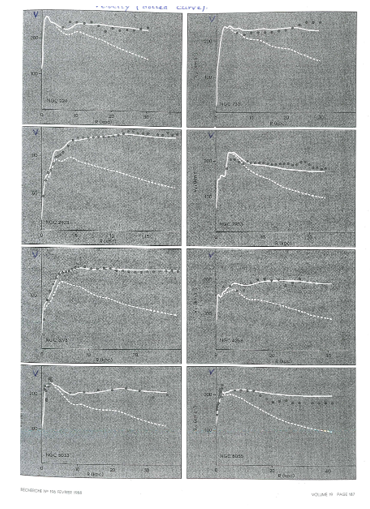Figure 2:  A Mac Laurin ellipsoid (oblate and homogeneous) Mass M; the three semi: axes a, a and b; eccentricity: e = [1 – (b²/a²)]1/2; F: focus; OF = ae ; A  = Arcsin eLet us consider first a homogeneous oblate ellipsoid of revolution, also called “Mac-Laurin ellipsoid” (fig 2), its inner gravitational potential Uin is the following in terms of its mass M, its density ρ, its semi-axes a and b, its eccentricity e (with b = a (1 – e²)1/2) and the cylindrical coordinates z (polar coordinate) and r (radial coordinate in the equatorial plane):(1)

with: G = constant of the law of Newton = 6.672. 10–11 m3/kg. s²                                     (2)

A = Arc sin e                                                                                          | (see figure 2)         (3)

F(e) = (A/e3) – [(1 – e²)1/2 /e²] = (2/3) + (e²/5) + (3e4/28) +…       |        ----                         (4)

H(e)= [1/ e²(1 – e²)1/2 ]  – (A/e3)                                                           |   ----                       (5)

With these expressions, the classical relation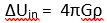is easy to verify: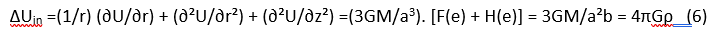The gravitational outer potential of homogeneous ellipsoids has a beautiful property: two homogeneous ellipsoids of the same mass, the same center, the same symmetries and the same focus in their symmetry planes have the same outer potential. Since the gravitational potential is a continuous function of the position, this property allows to know easily the outer potential, at least in the equatorial plane at an arbitrary distance r: consider a larger ellipsoid with semi major axis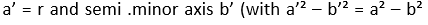for the common focus). This leads to: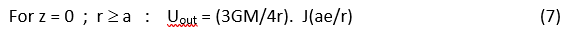the function J(ae/r) being the following with ae/r = x: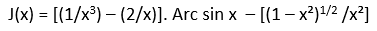(8)

that is, with (4):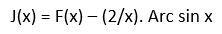(9)

The inner and outer potential give the “circular velocity” V, i.e., the velocity allowing to move freely along a circular orbit (z=0, ‘r’ constant) in the gravitational field of interest: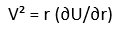(10)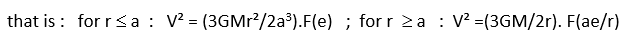(11)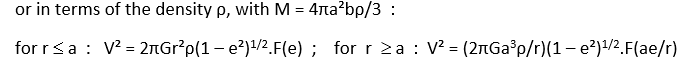(12)

We thus obtain the circular velocity V for a homogeneous elliptic galaxy, but the equation (10) tells us also that V² is a linear function of any addition, or subtraction, of arbitrary potentials with the same spheroidal symmetries (i.e., a symmetry about the equatorial plane and a symmetry of revolution about the polar axis). Of course, the circular velocities given by (11) or by (12) are very far from the observations (compare the figures 1 and 3): the elliptic galaxies are not homogeneous.

Figure 3: The circular velocity for a Mac Laurin ellipsoid (case e = 0.98; b/a ≈ 0.2)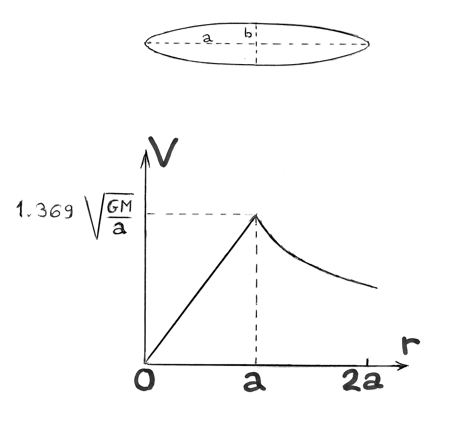However, these velocities lead to an excellent means of analysis of the dynamic of galaxies. Let us consider indeed in figure 4 the narrow space between the oblate ellipsoid of semi-axes a and b and the slightly larger ellipsoid with the same center, the same symmetries, the same eccentricity e and the semi-axes a+δa and b+δb (and henceδa/a = δb/b since the two eccentricities are equal). That narrow space can be considered as the difference between the two ellipsoids and a homogeneous mass δM of density ρ filling that space can be considered as the difference of the two ellipsoids with that same density. The difference of the expressions (11) or (12) for the corresponding ellipsoids give the influence of the mass δM on the circular velocity…

Figure 4:  A homogeneous layer between two similar and concentric ellipsoids Mass δM; semi-axes a and a + δa.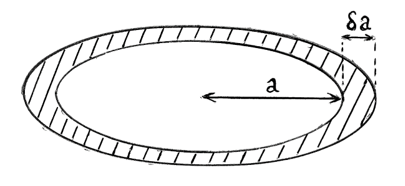The first expression of (12) shows easily that this difference is zero if r ≤ a, and the attraction of the mass dM on an inner point is zero (that is already well known if the eccentricity e is zero and if the two ellipsoids are two concentric spheres). For r ≥ a + δa , the computation is more difficult, we must derive the second expression of (12) with respect to the semi-major axis a, with constant e, ρ and r, fortunately the result is simple, the contribution of the mass δM on V² is  G.δM / ( r² – α²e²)1/2 where α is a length between a and a + δa.

Figure 5: Decomposition into successive homogeneous, concentric and similar layers.The next step is of course to divide an oblate ellipsoid into a series of concentric ellipsoids of all the same eccentricity e (figure 5), with the semi-major axis ‘a’ increasing from zero to some maximum value aM. If then in a layer between two neighboring ellipsoids the density is constant and if we call M(a) the mass included inside the ellipsoid of semi-major axis a, the circular velocity V(r) at the distance r in the equatorial plane is given by the following simple integral: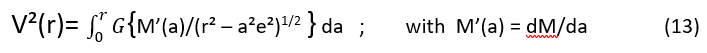A few examples

1. The case e = 0

In the case e = 0, the equation (13) gives directly: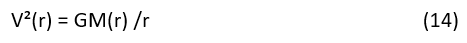This well-known equation, concerning systems with a spherical symmetry, shows how large is the possibility of choice of V(r) according to the repartition of the masses, the only conditions are A) The product V²r is non-decreasing, B) its final value is GM.

2.The case e = 0.98

The galaxies are not spherical and an eccentricity of 0.98 is more adapted than e = 0, it gives a ratio b/a almost equal to 0.2, that is more realistic. Let us look for a possible repartition of masses, with this eccentricity of 0.98, in a typical case close to the observations (see figure 6) a case in which the circular velocity is constant when r is larger than some limit L.

Figure 6: A typical example: a mass and density repartition when the circular velocity is constant for large r.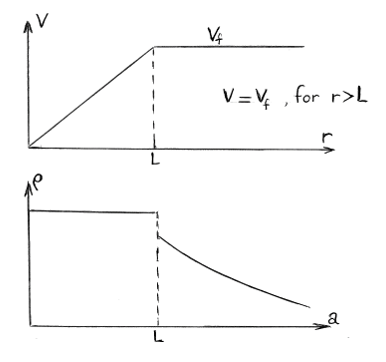If we choose a circular velocity proportional to r when r is smaller than the limit L, then the corresponding density is constant for all a < L; but after that limit the density is decreasing a little faster than 1/a².

Notice:

A) The case e = 0.98 has the same kind of freedom already encountered for e = 0, a freedom even a little larger (it requires less mass).

B) That freedom of choice of the repartition of masses for a given dynamic of a galaxy is even far larger since it is always possible to mix several repartitions corresponding to different values of the eccentricity e.

C) A decrease of the density in 1/a² doesn’t gives a decrease of the circular velocity V.

D) On a picture of a galaxy (a two-dimensional analysis) a decrease in 1/a² correspond to a decrease in 1/r2.5, that is a very fast decrease. Hence the decrease of the circular velocity V for large r will require much faster decreases of the density.

It is of course always possible to consider densities with a very fast decrease, faster than 1/r2.5, but this leads to another surprise, as in the following (see figure 7).

Figure 7: The circular velocity for a galaxy with a bulge.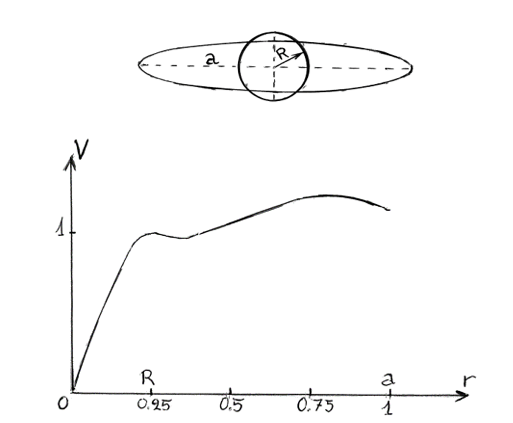We will consider the following repartition of mass:

A) An elliptic galaxy: an oblate ellipsoid of eccentricity e = 0.98 of semi-major axis a =1 with then b = semi-minor axis = a (1 – e²)1/2 = 0.198 99… and with the following density ρ: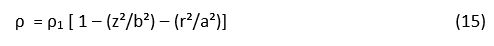The density is maximum at the center and zero at the surface of the ellipsoid. The corresponding mass is….B) To this ellipsoid we will add a spherical bulge with the same center and with a small radius R and a mass M2. That addition is not artificial: most galaxies have a central nearly spherical bulge with a mass that can be as large as 20% of the total mass of the galaxy. We will choose for that bulge a repartition of mass similar to (15):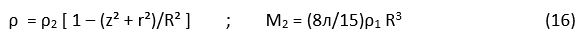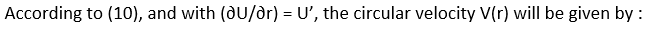(17)

where U1 and U2 are the gravitational potential of the masses M1 and M2 respectively.

All computations done, we will obtain, with A = Arcsin e and F(e) given in (4):that is with e = 0.98 and a = 1:Hence, if we consider the case where G = 1, M1 = 0.8, M2 = 0.2 and R = 0.25, the circular velocity is given by the following: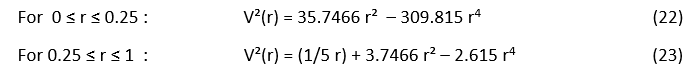The corresponding function V(r) is presented in figure 7 and it looks very much like the upper curves of figure 1 (especially the sixth one). The stars of this elliptic galaxy have a fast rotation and are in equilibrium.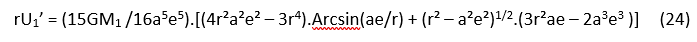with of course a = 1 and e = 0.98 in the present case (notice that the parameters a and e only appears through their product ae).

Figure 8: Extension of figure 7 for r > a.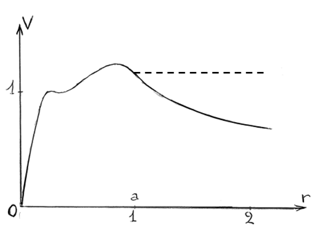The corresponding circular velocity V(r) defined byis the full curve presented in figure 8 and, for r > 1, it decreases usually much more rapidly than the observed velocities (dotted line), but how can we observe the velocities, since for r > 1 the density is zero? We observe the clouds of gas that extend much farther than the stars. This situation is very general: the “too fast rotation” observed in the motion of stars can be explained by a suitable repartition of the masses of the stars themselves, but very often the clouds of gas that are surrounding a galaxy have a velocity higher, and sometimes much higher, than the local circular velocity; and no suitable repartition of mass of the stars of the galaxy can erase that discrepancy…

The two classical explanations of this situation are those of references 1 - 3.  Either A) with Fritz Zwicky, S. Smith and Vera Robin, a large suitable quantity of invisible “Dark Matter” is supposed to lie at the suitable place inside and in the vicinity of the galaxy or B) with Mordehai Milgrom, a suitable correction of the law of gravitational attraction is assumed for very small attractions. But there is a third possibility: the fast-rotating outer clouds of gas are not in equilibrium; they are escaping into outer space.

We have a similar situation in the Solar System with the “solar wind” of several million tons per second of small particles continuously launched in space by the Sun and disappearing in interstellar space at velocities between 300 and 500 km/s, according to the solar activity. This small solar wind has probably existed since the birth of the Sun, its total mass during the life of the Sun is only about 0.03% solar mass and this doesn’t affect the equilibrium of the Solar System.

## About the clusters of galaxies

The dynamic of the clusters of galaxies is even more complex and strange, the “necessary” quantity of “Dark Matter” is sometimes ten times larger than that of visible matter… However, let us notice that in this dynamic the relativistic effects are not at all negligible as they are inside a galaxy.

Let consider for instance the Friedman-Lemaître metric in the “flat” case (i.e., the simplest case):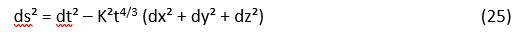x, y, z are the co-mobile coordinates in which the galaxies have small velocities, t is the usual time (with t = 0 at the big bang) and, if we wish that the co-mobile coordinates express presently the ordinary distances, K is a constant equal to 1 /cT2/3 (with c = velocity of light = = 3 × 108 m/s, and T = present age of Universe = 13.8 billion of years).

Let us consider now two massless particles at rest in the co-mobile system: their mutual distance increases with the expansion of Universe and is proportional to t2/3: that increase is not linear and there is a relative negative acceleration. If we extend this analysis to the motion of a system as a cluster of galaxies (a system in which the bodies have slow motions) the main relativistic effect is related to this negative acceleration: to the ordinary gravitational attraction, we must add a general acceleration directed toward the center of mass of the system and   proportional to the distance: for a vector ‘r’ that acceleration is (– 2r/9t²).

This relativistic acceleration is not at all negligible, it is of the order of magnitude of the gravitational accelerations inside the cluster and its centripetal direction is favorable to the stability of the cluster. Let us also notice the three following elements: A) This relativistic effect is proportional to 1/t² and was stronger in the past. B) The distant clusters are observed in a as they were in a distant past. C) The clusters can be in a state of slow dispersion, but that dispersion will take billions of years: the Andromeda galaxy is approaching with a velocity of 120 km/s but with that velocity it will require 6 billion of years before a collision with our galaxy…

Two galaxies, a front view and a side view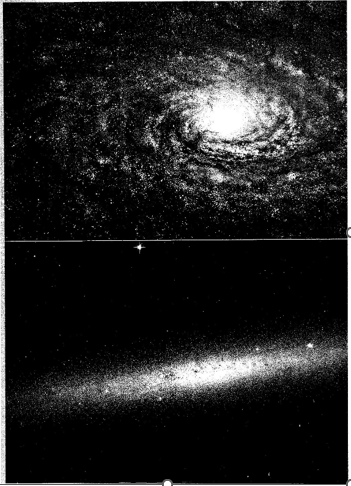## Conclusion

The mystery of the super rotation of galaxies can be at least partially solved without dark matter and without modification of the law of gravitation if full equilibrium is not required.  It is indeed possible to ensure the equilibrium of the stars, with a suitable repartition of their masses, if the equilibrium of the clouds of gas is not required: a part of the gas will escape to outer space. The equilibrium of clusters of galaxies is a more complex phenomenon requiring a full relativistic analysis and it seems that at least the clusters of galaxies can be stable during billions of years, even if their faraway future is dispersion.